# What is probability?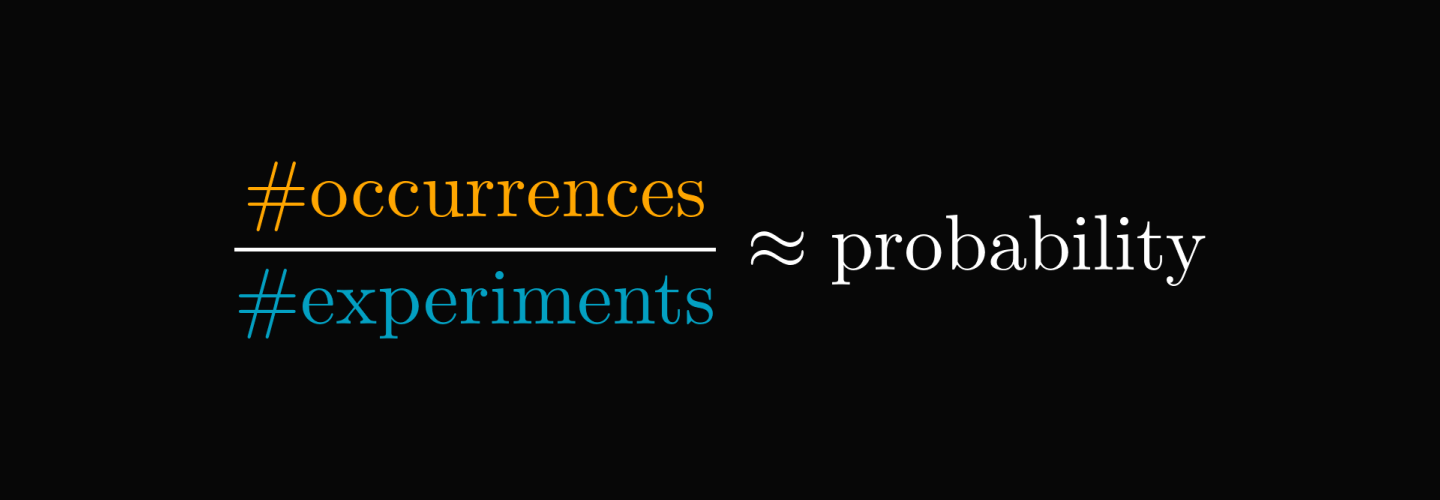You are (probably) wrong about probability.

I'll show you. How many heads you'll get if you toss a fair coin $10$ times?

If you answered $5$, here is why you are wrong.

(Below is the 100 HUF coin, if you were wondering.)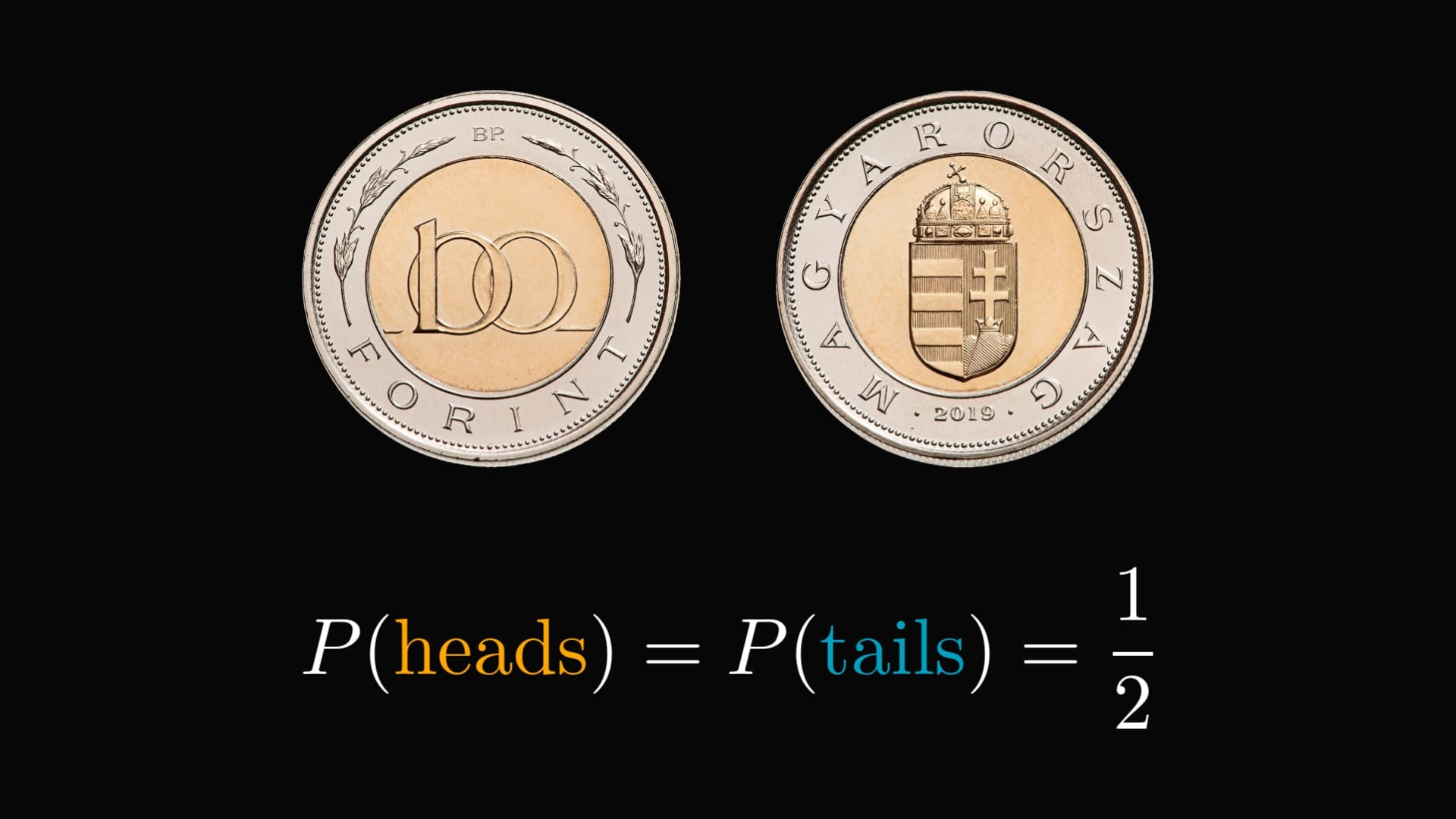You might as well try it if you have a coin in front of you.

All we can say with $100 \%$ certainty is that the number of heads will be between zero and ten.

However, that's not all to it.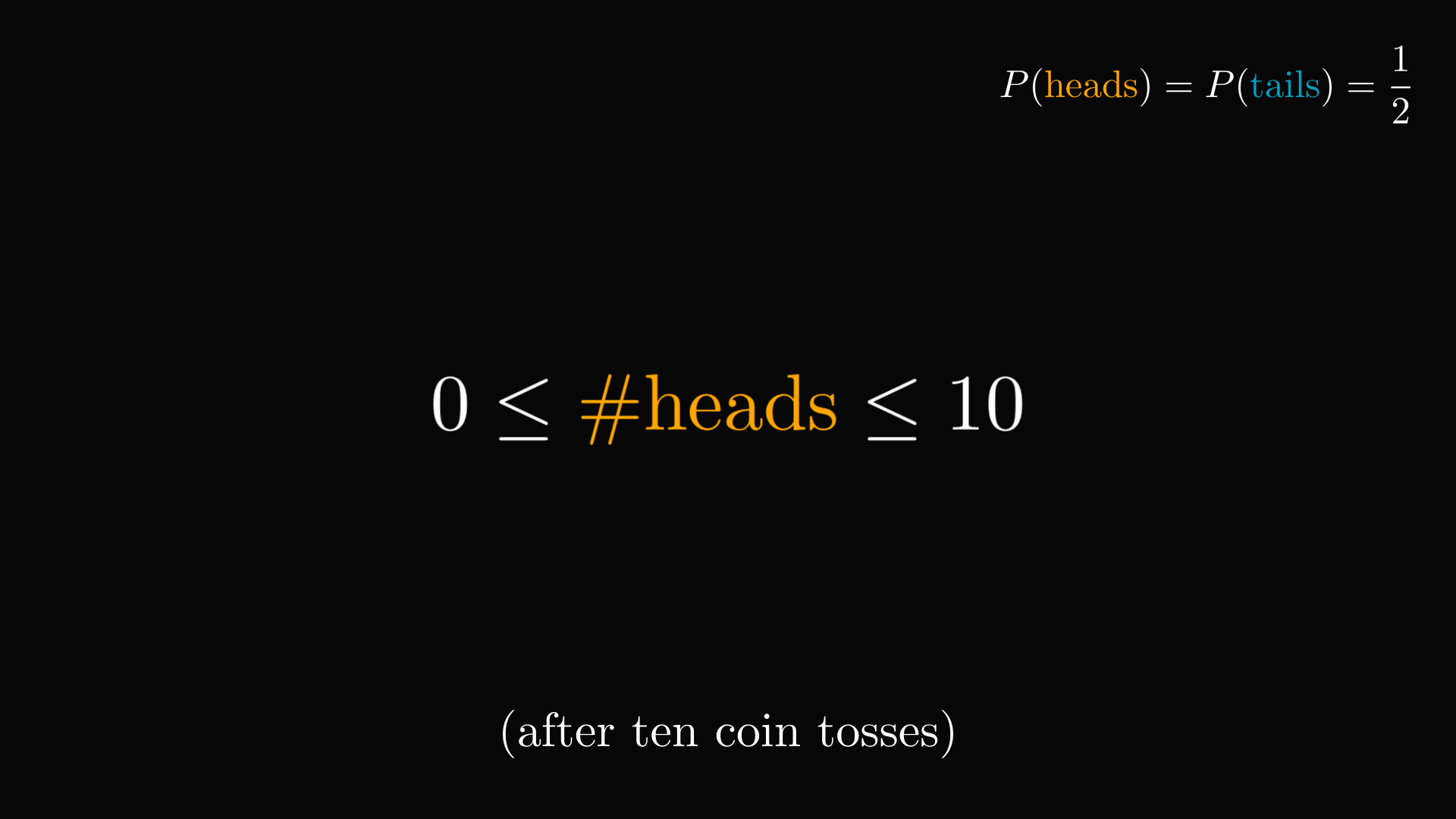For instance, the probability of landing $8$ heads and $2$ tails is approximately $4.39 \%$.

What does then the $1/2$ probability of heads mean?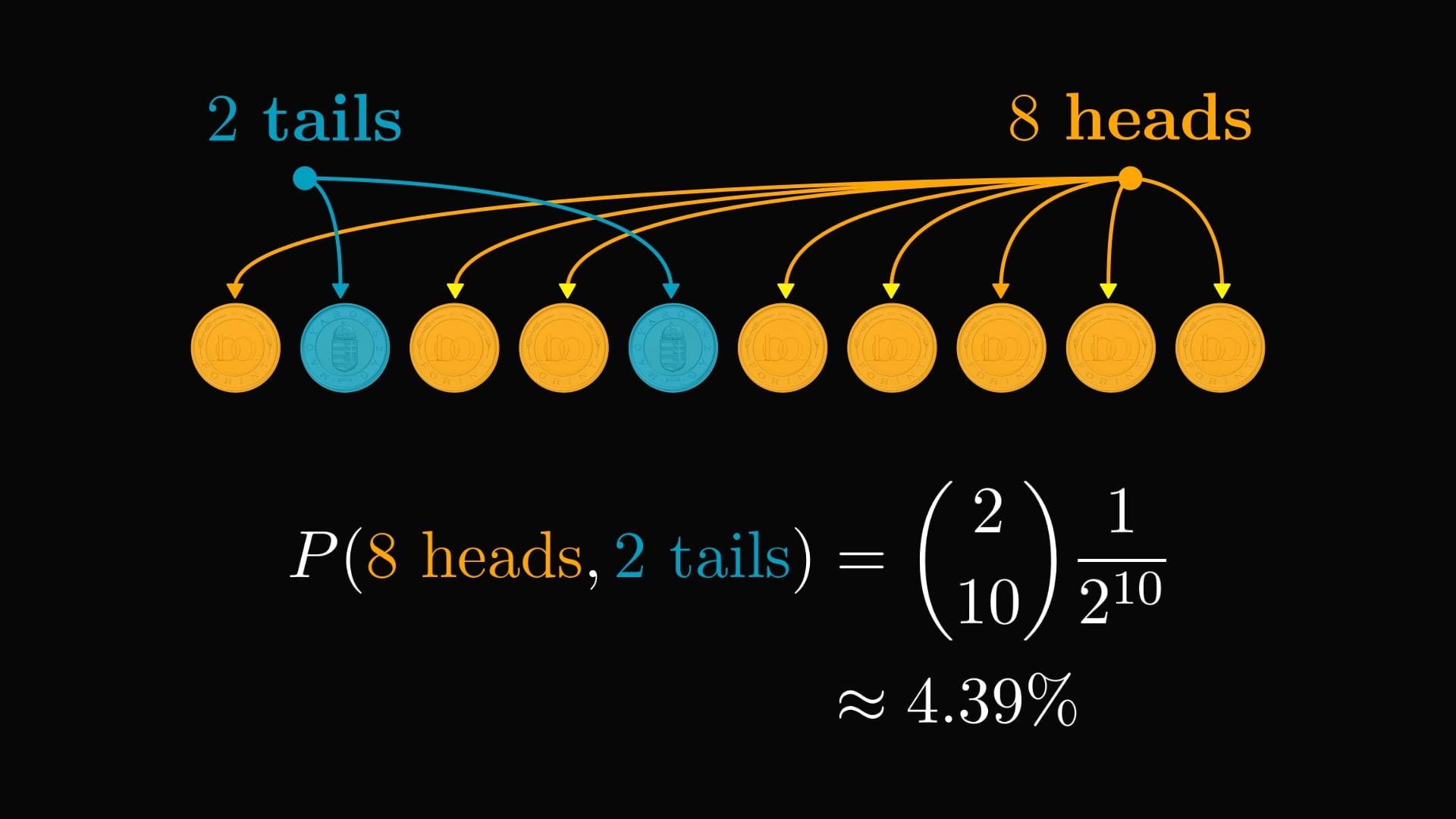Let's keep tossing the coin. Even though the number of heads and tails varies, it will fluctuate around $1/2$, that is, the probability of heads.

This is what probability represents.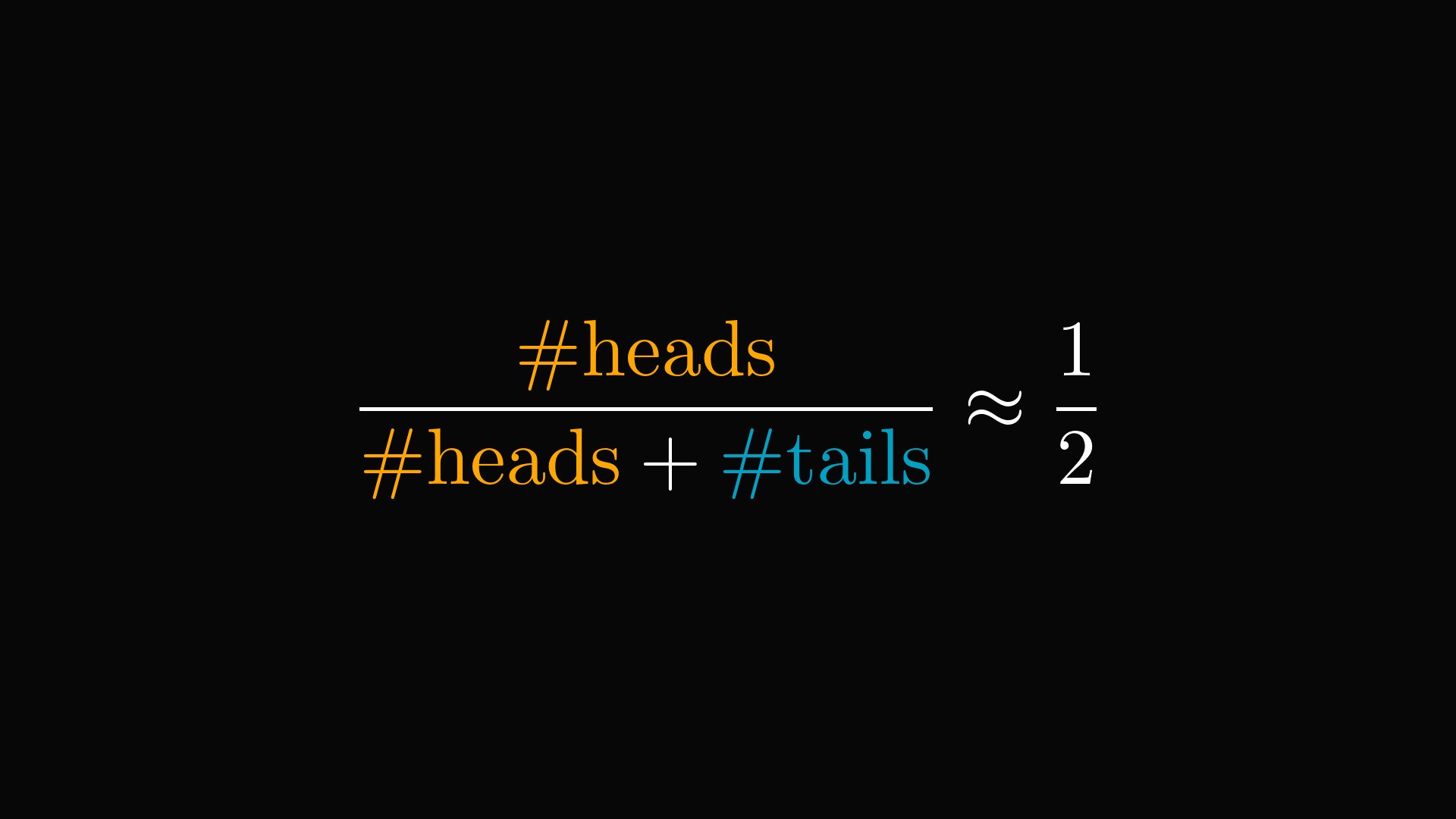In general, the relative frequency converges to the probability.

(The relative frequency is the ratio of occurrences of a given event and the number of experiments.)

This is the frequentist interpretation of probability.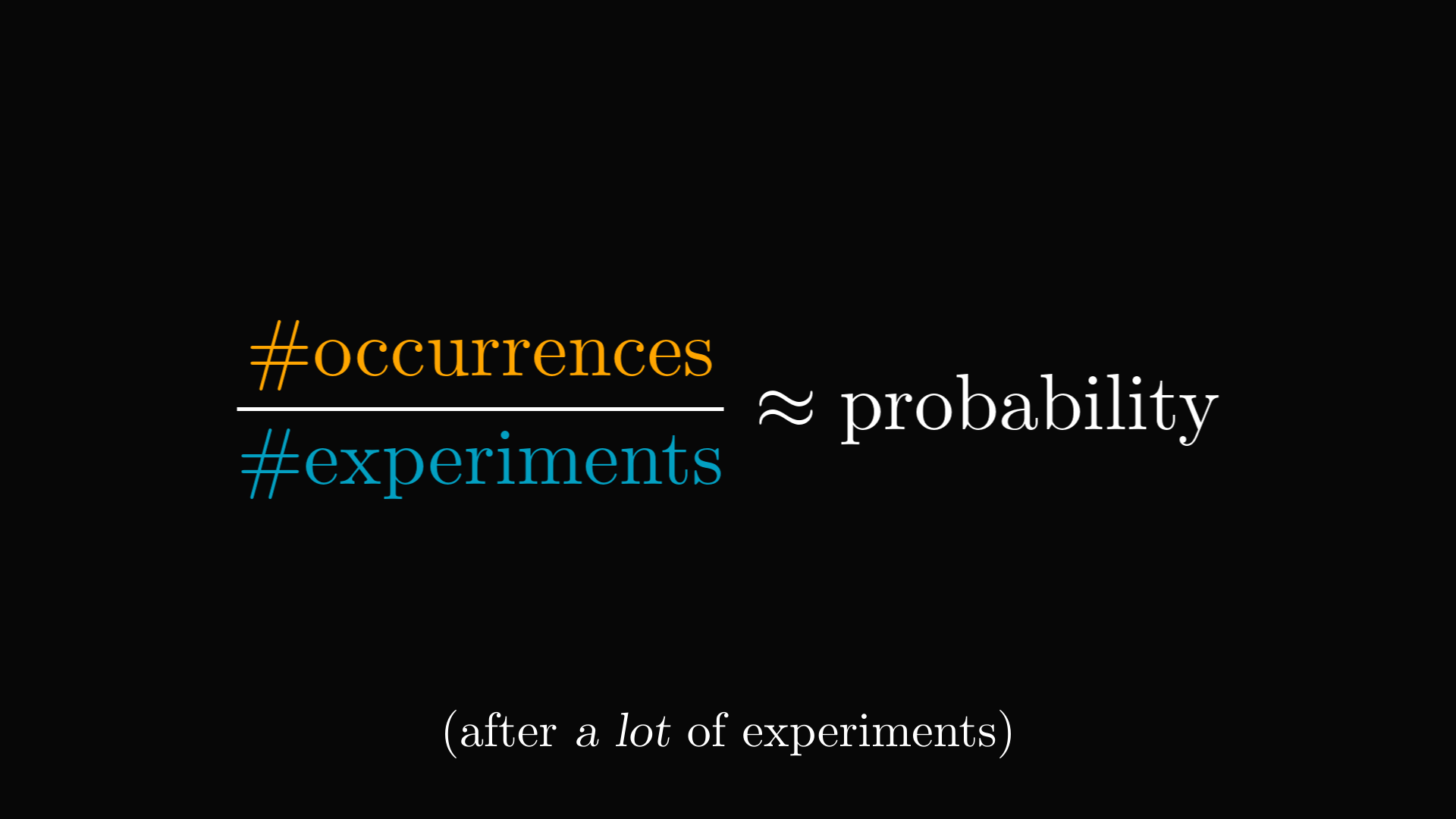The frequentist interpretation is only one side of the coin, so to speak. If you are curious about the full picture, check out my recent post about the frequentist and Bayesian interpretations!